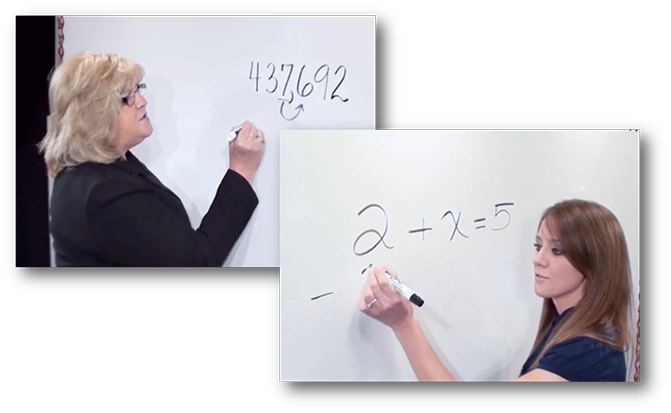Purposeful Moments in Math: VideosPurposeful Design Publications is pleased to provide a series of short video presentations - "Purposeful Moments in Math" - as a resource to aid teachers, parents and students in reviewing a variety of math operations, using a step by step process. We encourage you to link these videos to your own school’s website so it is convenient for students and parents to access these valuable tips.

These videos do not take the place of classroom instruction or the use of manipulatives and modeling in teaching concepts.

 Adding Two Integers with Different Signs  Adding Two Integers with the Same Sign Addition of Fractions with Like Denominators Addition of Fractions with Unlike Denominators Addition with Regrouping Changing Fractions to Decimals and Percents Compatible Numbers Dividing Fractions Dividing with Decimals Division of Large Numbers Finding an Average Finding Area and Volume Finding Perimeter Fractions in Simplest Form Greatest Common Factor Lattice Multiplication Least Common Denominator Multiplying and Dividing Mixed Integers Multiplying and Dividing Negative Integers Multiplying and Dividing Positive Integers Multiplying Decimals Multiplying Fractions and Cross Cancelling Multiplying Large Numbers Proportional Ratios Rounding Numbers Solving One Step Equations Subtracting Integers Subtracting with Decimals Subtraction of Mixed Numbers Subtracting with RegroupingFor additional information or to learn about a 60-day preview copy, contact your Educational Resource Consultant (ERC).